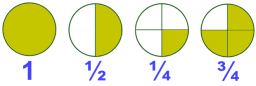# Value of Z

For x = -9, what is the value of Z, where Z equals fraction numerator x minus 17 over denominator 6.5 end fraction

Z =  -4

### Step-by-step explanation:Did you find an error or inaccuracy? Feel free to write us. Thank you!

Tips for related online calculators
Need help calculating sum, simplifying, or multiplying fractions? Try our fraction calculator.